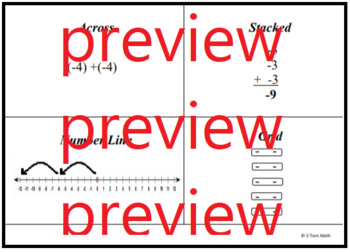# 7.NS.2 Modeling Multiplication of Negatives as Repeated AdditionSubject
Resource Type
File Type

PDF

(522 KB|12 pages)
Standards
Also included in:
1. Start students off confidently with the 3 Tiers original Stack, Add or Subtract, Leader of the pack adding model. Then work through subtraction, absolute value, multiplication, and division, engaging fractions decimals and word problems throughout. Includes bonus set of flashcard / notes hybrid th
\$13.00
\$9.00
Save \$4.00
• Product Description
• StandardsNEW

This introduction to multiplying negatives has students sort cards of repeated addition of negatives on a number line, a grid, stacked, and across, and identifying them as a multiplication problem. After they are sorted, students make the models for the cards that did not have a match.

Students extend their understanding using the commutative property of multiplication and create real life expressions that model repeated addition of negatives as multiplication. Finally, students try multiplication with fractions and decimals - but beware - addition and subtraction problems are scattered throughout to keep their minds sharp! Unpacking the concept of a negative times a negative is tackled in the next lesson, Strings of Multiplication (Neutralizing Negatives 9).

About the 3 TIERS MATH model:

We have analyzed and targeted the known struggles for this topic by scaffolding the lesson in 3 differentiated versions: Independent, Hints, and Guided. Word banks, answer keys, sentence starters, templates, hints, problem chunking and other strategies are used to provide a means for less confident students access the same curriculum and rigor, allowing all students to engage in one common learning experience.

This lesson is designed for students to work through in pairs or groups to discover the big ideas through guided inquiry. While some direct instruction is required, the structure empower students to be independent learners, where your role transitions to more of a mentor than teacher. It is the eighth lesson in the Neutralizing Negatives Unit for a 7th grade Common Core math class. There is a different lesson that is geared toward a 7th grade Accelerated Math class (not included).

Apply properties of operations as strategies to multiply and divide rational numbers.
Understand that multiplication is extended from fractions to rational numbers by requiring that operations continue to satisfy the properties of operations, particularly the distributive property, leading to products such as (–1)(–1) = 1 and the rules for multiplying signed numbers. Interpret products of rational numbers by describing real-world contexts.
Apply and extend previous understandings of multiplication and division and of fractions to multiply and divide rational numbers.
Total Pages
12 pages
Included
Teaching Duration
50 minutes
Report this Resource to TpT
Reported resources will be reviewed by our team. Report this resource to let us know if this resource violates TpT’s content guidelines.